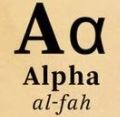D

#### Serena is in a math class of 𝟐𝟎 students. Each day for a week (Monday to Friday), a student in Serena’s class is randomly selected by the teacher to explain a homework problem. Once a student’s name is selected, that student is not eligible to be selected again that week.

45 viewed last edited 2 years agoAnonymous
0
1. What is the probability that Serena is selected on Monday?
2. What is the probability that Serena is selected on Tuesday given that she was not selected on Monday?
3. What is the probability that she will be selected on Friday given that she was not selected on any of the other days?Anuj Gupta
0

Recall that probability of an event happening is P(E) = number of favorable outcomes/ total number of outcomes.

1. So, the probability of selecting 1 student from a class of 20 students on a Monday is \frac{1}{20} = 0.05 .
2. Since once a student is selected, that student is not eligible to be selected again that week, there are 19 students left, and the probability that Serena is selected among those 19 students is \frac{1}{19} = 0.0526.
3. Similarly there will be 16 students left on Friday, so the probability that Serena is selected among these 16 students is \frac{1}{16} = 0.0625.